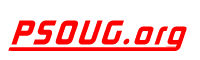Quick Search: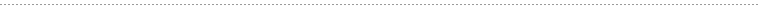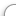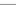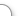CODE Oracle PL/SQL Code Library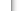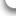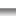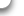JOBS Find Or Post Oracle JobsFORUM Oracle Discussion & ChatPHP Code: Gradient Image Generator Jump to: Select a Location PSOUG Home Page Oracle Code Library mySQL Code Library PHP Code Library JavaScript Code Library Oracle Terms & Definitions Oracle Error Codes PSOUG Community Blogs Oracle Jobs Board PSOUG Forum Oracle User Group Directory Free Oracle Magazines Online Learning Center PSOUG Presentations Advanced Code Search News and Events Sponsors Page Submit Code Contact UsDescription: The default gradient is set at black->white. To change it, just add the start and end variables.

Example usage:

Comment: (none)

Language: PHP
Highlight Mode: PHP

```<?PHP

\$height = 100;
\$width = 50;

\$start = '000000';
\$end = 'FFFFFF';
IF (\$_GET["start"]) {
\$start = \$_GET["start"];
}
IF (\$_GET["end"]) {
\$end = \$_GET["end"];
}

\$start_r = HEXDEC(SUBSTR(\$start, 0, 2));
\$start_g = HEXDEC(SUBSTR(\$start, 2, 2));
\$start_b = HEXDEC(SUBSTR(\$start, 4, 2));
\$end_r = HEXDEC(SUBSTR(\$end, 0, 2));
\$end_g = HEXDEC(SUBSTR(\$end, 2, 2));
\$end_b = HEXDEC(SUBSTR(\$end, 4, 2));
\$image = @imagecreate(\$width, \$height);

FOR(\$y=0;\$y<\$height;\$y++) {
FOR(\$x=0;\$x<\$width;\$x++) {
IF (\$start_r == \$end_r) {
\$new_r = \$start_r;
}
\$difference = \$start_r - \$end_r;
\$new_r = \$start_r - INTVAL((\$difference / \$height) * \$y);
IF (\$start_g == \$end_g) {
\$new_g = \$start_g;
}
\$difference = \$start_g - \$end_g;
\$new_g = \$start_g - INTVAL((\$difference / \$height) * \$y);
IF (\$start_b == \$end_b) {
\$new_b = \$start_b;
}
\$difference = \$start_b - \$end_b;
\$new_b = \$start_b - INTVAL((\$difference / \$height) * \$y);
\$row_color = imagecolorresolve(\$image, \$new_r, \$new_g, \$new_b);
imagesetpixel(\$image, \$x, \$y, \$row_color);
}
}

imagepng(\$image);
imagedestroy(\$image);

// Example usage:

?>```FreeOracle MagazineSubscriptionsand Oracle White Papers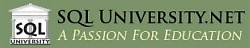SQL University.net courses meet the most demanding needs of the business world for advanced education in a cost-effective manner. SQL University.net courses are available immediately for IT professionals and can be taken without disruption of your workplace schedule or processes.

Compared to traditional travel-based training, SQL University.net saves time and valuable corporate resources, allowing companies to do more with less. That's our mission, and that's what we deliver.